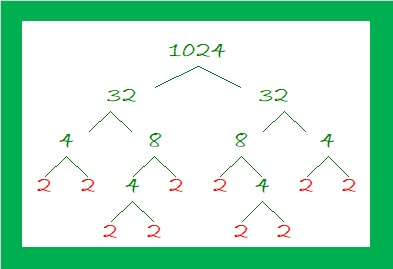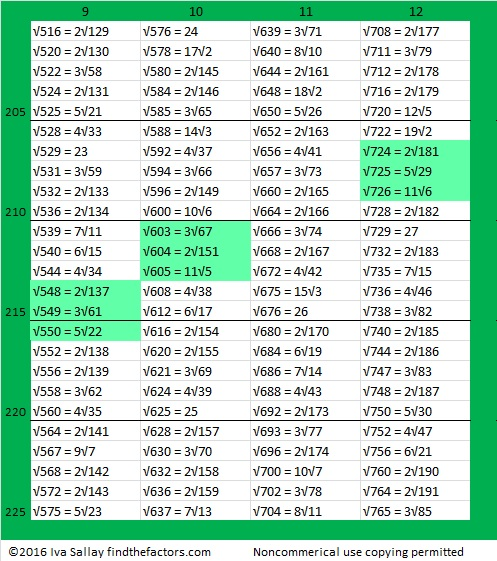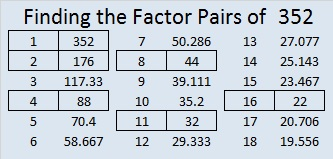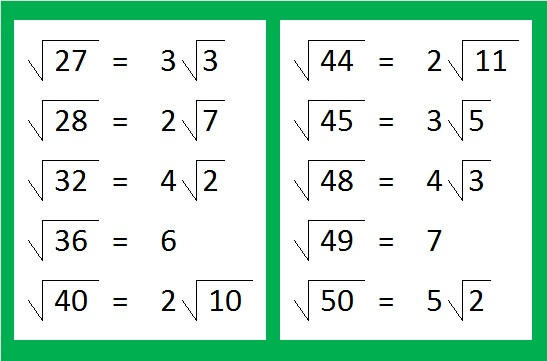What’s special about √1024? Is it because it and several counting numbers after it have square roots that can be simplified?Perhaps.

Maybe it is interesting just because √1024 = 32, a whole number. The 5th root of 1024 = 4 and the 10th root of 1024 = 2, both whole numbers as well.

Those equations are true because 32² = 1024, 4⁵ = 1024, and 2¹⁰ = 1024.

Or perhaps 1024 is special because it is the smallest number that is a 10th power. (It is 2¹⁰.) The square root of a perfect 10th power is always a perfect 5th power. (32 = 2⁵ and is the smallest number that is a 5th power.)

1024 is also the smallest number with exactly 11 factors.

It is the smallest number whose factor tree has at least 10 leaves that are prime numbers. (They are the red leaves on the factor tree shown below.) It is possible to draw several other factor trees for 1024, but they will all have the number 2 appearing ten times.What’s more, I noticed something about 1024 and some other multiples of 256: Where do multiples of 256 fall on the list of square roots that can be simplified?

• 256 × 1 = 256 and 256 is the 100th number on this list of numbers whose square roots can be simplified.• 256 × 2 = 512. When we add the next 100 square roots that can be simplified, 512 is the 199th number on the list.• Here are the third 100 square roots that can be simplified:• 256 × 3 = 768 didn’t quite make that list because it is the 301st number. Indeed, it is the first number on this list of the fourth 100 numbers whose square roots can be simplified.• 256 × 4 = 1024. That will be the first number on the 5th 100 square roots list!

It is interesting that those multiples of 256 have the 100th, the 199th, the 301st, and the 401st positions on the list. That is so close to the 100th, 200th, 300th, and 400th positions.

In case you couldn’t figure it out, the highlighted square roots are three or more consecutive numbers that appear on the list.

1024 is interesting for many other reasons. Here are a few of them:

(4-2)¹⁰ = 1024, making 1024 the 16th Friedman number.

I like to remember that 2¹⁰ = 1024, which is just a little bit more than a thousand. Likewise 2²⁰ = 1,048,576 which is about a million. 2³⁰ is about a billion, and 2⁴⁰ is about a trillion.

*******
As stated in the comments, Paula Beardell Krieg shared a related post with me. It takes exactly 1024 Legos to build this fabulous pyramidal fractal:

1024 has so many factors that are divisible by 4 that it is a leg in NINE Pythagorean triples:
768-1024-1280 which is (3-4-5) times 256
1024-1920-2176 which is (8-15-17) times 128
1024-4032-4160 which is (16-63-65) times 64
1024-8160-8224 which is (32-255-257) times 32
1024-16368-16400 which is 16 times (64-1023-1025)
1024-32760-32776 which is 8 times (128-4095-4097)
1024-65532-65540 which is 4 times (256-16383-16385)
1024-131070-131074 which is 2 times (512-65535-65537),
and primitive 1024-262143-262145

Some of those triples can also be found because 1024 is the difference of two squares four different ways:
257² – 255² = 1024
130² – 126² = 1024
68² – 60² = 1024
40² – 24² = 1024
To find out which difference of two squares go with which triples, add the squares instead of subtracting and you’ll get the hypotenuse of the triple.
******

1024 looks interesting in some other bases:
It’s 1000000000 in BASE 2,
100000 in BASE 4,
2000 in BASE 8,
1357 in BASE 9,
484 in BASE 15,
400 in BASE 16,
169 in BASE 29,
144 in BASE 30,
121 in BASE 31, and
100 in BASE 32

• 1024 is a composite number.
• Prime factorization: 1024 = 2 × 2 × 2 × 2 × 2 × 2 × 2 × 2 × 2 × 2, which can be written 1024 = 2¹⁰
• The exponent in the prime factorization is 10. Adding one we get (10 + 1) = 11. Therefore 1024 has exactly 11 factors.
• Factors of 1024: 1, 2, 4, 8, 16, 32, 64, 128, 256, 512, 1024
• Factor pairs: 1024 = 1 × 1024, 2 × 512, 4 × 256, 8 × 128, 16 × 64, or 32 × 32,
• 1024 is a perfect square. √1024 = 32. It is also a perfect 5th power, and a perfect 10th power.# 783 and Level 2

• 783 is a composite number.
• Prime factorization: 783 = 3 x 3 x 3 x 29, which can be written 783 = (3^3) x 29
• The exponents in the prime factorization are 3 and 1. Adding one to each and multiplying we get (3 + 1)(1 + 1) = 4 x 2 = 8. Therefore 783 has exactly 8 factors.
• Factors of 783: 1, 3, 9, 27, 29, 87, 261, 783
• Factor pairs: 783 = 1 x 783, 3 x 261, 9 x 87, or 27 x 29
• Taking the factor pair with the largest square number factor, we get √783 = (√9)(√87) = 3√87 ≈ 27.982137.Here’s today’s puzzle. It’s a level 2 so it isn’t very difficult:Print the puzzles or type the solution on this excel file: 12-factors-782-787

—————————————

27 x 29 = 783. Since (n – 1)(n + 1) always equals n² – 1, we know that 783 is one number away from the next perfect square.

29 is a factor of 783, making 783 the hypotenuse of a Pythagorean triple:

• 540-567-783, which is 27 times 20-21-29.
• Thus 540² + 567² = 783² just as 20² + 21² = 29².

783 is also a palindrome in bases 15, 23, and 28:

• 373 BASE 15; note that 3(225) + 7(15) + 3(1) = 783
• 1B1 BASE 23 (B is 11 base 10); note that 1(23²) + 11(23) + 1(1) = 783
• RR BASE 28 (R is 27 base 10); note that 27(28) + 27 = 783

—————————————# 456 An Inchworm Measuring Marigolds

456 is the sum of consecutive prime numbers in two different ways. One of my readers listed those ways in the comments. The factors of 456 are at the end of the post.Inchworm, inchworm,
Measuring the marigolds
You and your arithmetic will probably go far.

Two plus two is four
Four plus four is eight
Eight and eight is sixteen
Sixteen and sixteen is thirty-two.

Inchworm, inchworm,
Measuring the marigolds
Seems to me you’d stop and see
How beautiful they are.

Today I taught a class of three year olds about being thankful for birds, insects, and creeping things. To keep their attention, I used a variety of stories, riddles, books, and games. I also sang a few songs including this one about an inchworm who is very good at arithmetic. I think preschool children can still enjoy songs like this even if they don’t understand everything the song is about or even if they are wiggling as much as an inchworm while they listen to it. Here is the song sung by Danny Kaye from the movie Hans Christian Andersen:

———————————————————————————————————

Now for the number 456. The last two digits can be evenly divided by four, so the entire number is divisible by four. Also since it is formed from three consecutive numbers, it is divisible by 3. However since the number in the middle of those consecutive numbers is not 3, 6, 9 or another multiple of 3, we know that 456 is NOT divisible by 9.

Because it is divisible by four, we will use that fact first to determine how to reduce its square root.456 ÷ 4 = 114. Notice that 114 is even, but 14 can’t be evenly divided by 4, so 114 cannot be either. Also notice that 114 is still divisible by 3. If we’re not sure whether or not 114 has any square factors, we are less likely to make a mistake if we divide it by 6 once, instead of by 2 and then by 3.114 ÷ 6 = 19, a prime number, and we are certain there were no other square factors. Since we know 19 x 6 = 114, let’s backtrack a little and go back to that original one layer cake:Take the square root of everything on the outside of the cake and get √456 = (√4)(√114) = 2√114

———————————————————————————————————

• 456 is a composite number.
• Prime factorization: 456 = 2 x 2 x 2 x 3 x 19, which can be written 456 = (2^3) x 3 x 19
• The exponents in the prime factorization are 3, 1, and 1. Adding one to each and multiplying we get (3 + 1)(1 + 1)(1 + 1) = 4 x 2 x 2 = 16. Therefore 456 has exactly 16 factors.
• Factors of 456: 1, 2, 3, 4, 6, 8, 12, 19, 24, 38, 57, 76, 114, 152, 228, 456
• Factor pairs: 456 = 1 x 456, 2 x 228, 3 x 152, 4 x 114, 6 x 76, 8 x 57, 12 x 38, or 19 x 24
• Taking the factor pair with the largest square number factor, we get √456 = (√4)(√114) = 2√114 ≈ 21.3542———————————————————————————————————

Picture credits: Inchworm and ruler: http://www.kindergartenkindergarten.com/2012/06/problem-solving-measurement.html;

# Reducible Square Roots of Numbers up to 352

• 352 is even and therefore a composite number.
• Prime factorization: 352 = 2 x 2 x 2 x 2 x 2 x 11, which can be written 352 = (2^5) x 11
• The exponents in the prime factorization are 5 and 1. Adding one to each and multiplying we get (5 + 1)(1 + 1) = 6 x 2 = 12. Therefore 352 has exactly 12 factors.
• Factors of 352: 1, 2, 4, 8, 11, 16, 22, 32, 44, 88, 176, 352
• Factor pairs: 352 = 1 x 352, 2 x 176, 4 x 88, 8 x 44, 11 x 32, or 16 x 22
• Taking the factor pair with the largest square number factor, we get √352 = (√16)(√22) = 4√22 ≈ 18.762Mathematics is full of interesting patterns. Let’s explore some patterns in reducible square roots.

Here are the first ten numbers that have reducible square roots. Notice that five of the numbers in this list, 1, 4, 9, 16, and 25 are perfect squares:40% of the numbers up to 5 have reducible square roots. The same thing is true for 40% of the numbers up to 10, 40% of the numbers up to 20, and 40% of the numbers up to 25.

Here are the second ten numbers with reducible square roots. The last number,50, is double the previous last number, 25. Again 40% of the numbers up to 50 have reducible square roots, and only two of these numbers are perfect squares. Notice that the last three numbers under the radical sign in this set are consecutive numbers.Would you like to make any predictions for the third set of ten numbers with reducible square roots?

If you predicted that this set of numbers would end with 75, you were almost right! 29/75 or 38.67% of the numbers up to 75 have reducible square roots, and 30/76 or 39.47% of the numbers up to 76 do. Both of these values are very close to 40%, but not quite there. Notice this time only one number is a perfect square.Would you like to make a prediction for what will happen with the fourth set of ten numbers with reducible square roots?

Surprise! We’re back to 40% of the numbers up to 100 have reducible square roots. Those consecutive numbers at the end of the set really helped raise the percentage right at the last minute. This set of numbers has two perfect squares.What do you think will happen if we look at all the reducible square roots up to 350? Multiples of any perfect square will always have reducible square roots. Since we have more perfect squares, do you think more will be reducible?

Because the square roots of 351 and 352 are also reducible, let’s include them in this chart. Each column has 20 reducible square roots in it, and they are grouped into fives for easier counting. I’ve highlighted sets of three or four consecutive numbers. In all, we now have charts showing the first 140 reducible square roots. This last set has eight perfect squares. Let’s look at the percentages at the end of some of those sets of consecutive numbers: 50/126 or 39.68% of the first 126 numbers have reducible square roots. 96/245 or 39.18% of the first 245 numbers have reducible square roots. Finally, 140/352 or 39.77% of the numbers up to 352 have reducible square roots. That one is so close to 40%!

No matter how big a table we make, the percentage of reducible square roots will be very close to 40%. It will not get significantly higher because, believe it or not, most numbers are either prime numbers or the product of two or more DIFFERENT primes and thus have square roots that are NOT reducible.